SPSS TUTORIALS BASICS ANOVA REGRESSION FACTOR CORRELATION

# SPSS Multiple Regression Analysis Tutorial

Running a basic multiple regression analysis in SPSS is simple. For a thorough analysis, however, we want to make sure we satisfy the main assumptions, which are

• linearity: each predictor has a linear relation with our outcome variable;
• normality: the prediction errors are normally distributed in the population;
• homoscedasticity: the variance of the errors is constant in the population.

Furthermore, let's make sure our data -variables as well as cases- make sense in the first place. Last, there's model selection: which predictors should we include in our regression model?
In short, a solid analysis answers quite some questions. So which steps -in which order- should we take? The table below proposes a simple roadmap.

StepWhy?Action
1Inspect histogramsSee if distributions make sense.Set missing values.
Exclude variables.
2Inspect descriptivesSee if any variables have low N.
Inspect listwise valid N.
Exclude variables with low N.
3Inspect scatterplotsSee if relations are linear.
Look for influential cases.
Exclude cases if needed.
Transform predictors if needed.
4Inspect correlation matrixSee if Pearson correlations make sense.Inspect variables with unusual correlations.
5Regression I: model selectionSee which model is good.Exclude variables from model.
6Regression II: residualsInspect residual plots.Transform variables if needed.

## Case: Employee Satisfaction Study

A company held an employee satisfaction survey which included overall employee satisfaction. Employees also rated some main job quality aspects, resulting in work.sav.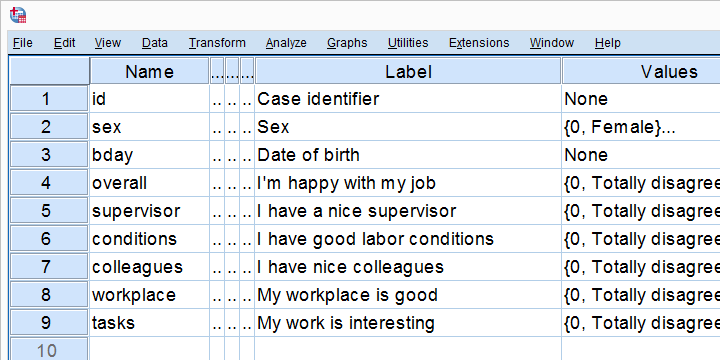The main question we'd like to answer is which quality aspects predict job satisfaction and to which extent? Let's follow our roadmap and find out.

## Inspect All Histograms

Right, before doing anything whatsoever with our variables, let's first see if they make any sense in the first place. We'll do so by running histograms over all predictors and the outcome variable. This is a super fast way to find out basically anything about our variables. Running the syntax below creates all of them in one go.

*Check histograms of outcome variable and all predictors.

/format notable
/histogram.

## ResultJust a quick look at our 6 histograms tells us that

If histograms do show unlikely values, it's essential to set those as user missing values before proceeding with the next step.

## Inspect Descriptives Table

If variables contain any missing values, a simple descriptives table is a fast way to evaluate the extent of missingness. Our histograms show that the data at hand don't contain any missings. For the sake of completeness, let's run some descriptives anyway.

*Check descriptives.

## Result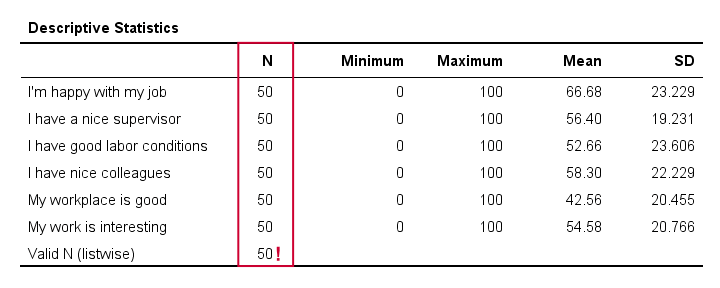The descriptives table tells us if any variable(s) contain high percentages of missing values. If this is the case, you may want to exclude such variables from analysis.
Valid N (listwise) is the number of cases without missing values on any variables in this table. By default, SPSS regression uses only such complete cases -unless you use pairwise deletion of missing values (which I usually recommend).

## Inspect Scatterplots

Do our predictors have (roughly) linear relations with the outcome variable? Basically all textbooks suggest inspecting a residual plot: a scatterplot of the predicted values (x-axis) with the residuals (y-axis) is supposed to detect non linearity. However, I think residual plots are useless for inspecting linearity. The reason is that predicted values are (weighted) combinations of predictors. So what if just one predictor has a curvilinear relation with the outcome variable? This curvilinearity will be diluted by combining predictors into one variable -the predicted values.
I think it makes much more sense to inspect linearity for each predictor separately. A minimal way to do so is running scatterplots of each predictor (x-axis) with the outcome variable (y-axis).
A simple way to create these scatterplots is to just one command from the menu. For details, see SPSS Scatterplot Tutorial. Next, remove all line breaks, copy-paste it and insert the right variable names as shown below.

*Inspect scatterplots all predictors (x-axes) with outcome variable (y-axis).

GRAPH /SCATTERPLOT(BIVAR)= supervisor     WITH overall /MISSING=LISTWISE.
GRAPH /SCATTERPLOT(BIVAR)= conditions     WITH overall /MISSING=LISTWISE.
GRAPH /SCATTERPLOT(BIVAR)= colleagues     WITH overall /MISSING=LISTWISE.
GRAPH /SCATTERPLOT(BIVAR)= workplace     WITH overall /MISSING=LISTWISE.
GRAPH /SCATTERPLOT(BIVAR)= tasks     WITH overall /MISSING=LISTWISE.

## ResultNone of our scatterplots show clear curvilinearity. However, we do see some unusual cases that don't quite fit the overall pattern of dots. We can easily inspect such cases if we flag them with a (temporary) new variable.

*Flag unusual case(s) that have (overall satisfaction > 40) and (supervisor < 10).

compute flag1 = (overall > 40 and supervisor < 10).

*Move unusual case(s) to top of file for visual inspection.

sort cases by flag1(d).

## Result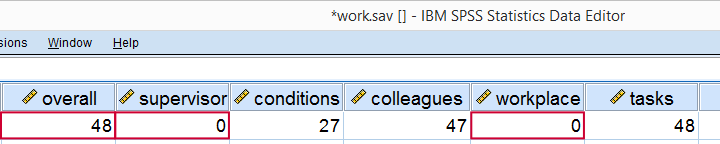Case (id = 36) looks odd indeed: supervisor and workplace are 0 (couldn't be worse) but overall job rating is not too bad. We should perhaps exclude such cases from further analyses with FILTER. But for now, we'll just ignore them.
Regarding linearity, our scatterplots provide a minimal check. For a more thorough inspection, try the excellent regression variable plots extension.The regression variable plots can quickly add some different fit lines to the scatterplots. This may clear things up fast.
A third option for investigating curvilinearity (for those who really want it all -and want it now) is running CURVEFIT on each predictor with the outcome variable.

## Inspect Correlation Matrix

We'll now see if the (Pearson) correlations among all variables (outcome variable and predictors) make sense. For details, see SPSS Correlation Analysis. For the data at hand, I expect only positive correlations between, say, 0.3 and 0.7 or so.

*Inspect if correlation matrix makes sense.

/print nosig
/missing pairwise.

## Result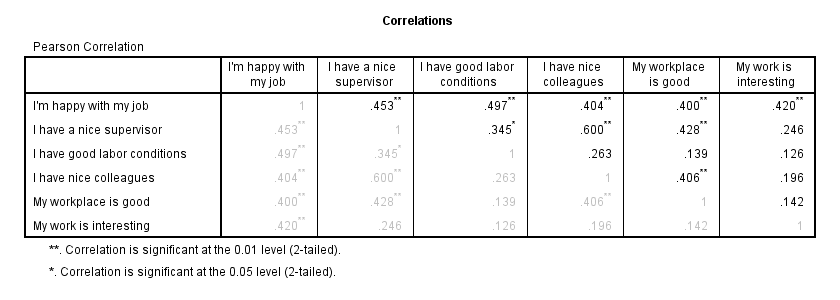The pattern of correlations looks perfectly plausible. Creating a nice and clean correlation matrix like this is covered in SPSS Correlations in APA Format.

## Regression I - Model Selection

The next question we'd like to answer is: which predictors contribute substantially to predicting job satisfaction? Our correlations show that all predictors correlate statistically significantly with the outcome variable. However, there's also substantial correlations among the predictors themselves. That is, they overlap. Some variance in job satisfaction accounted by a predictor may also be accounted for by some other predictor. If so, this other predictor may not contribute uniquely to our prediction.
There's different approaches towards finding the right selection of predictors. One of those is adding all predictors one-by-one to the regression equation. Since we've 5 predictors, this will result in 5 models. So let's see what happens. We'll navigate to AnalyzeRegressionLinear and fill out the dialog as shown below.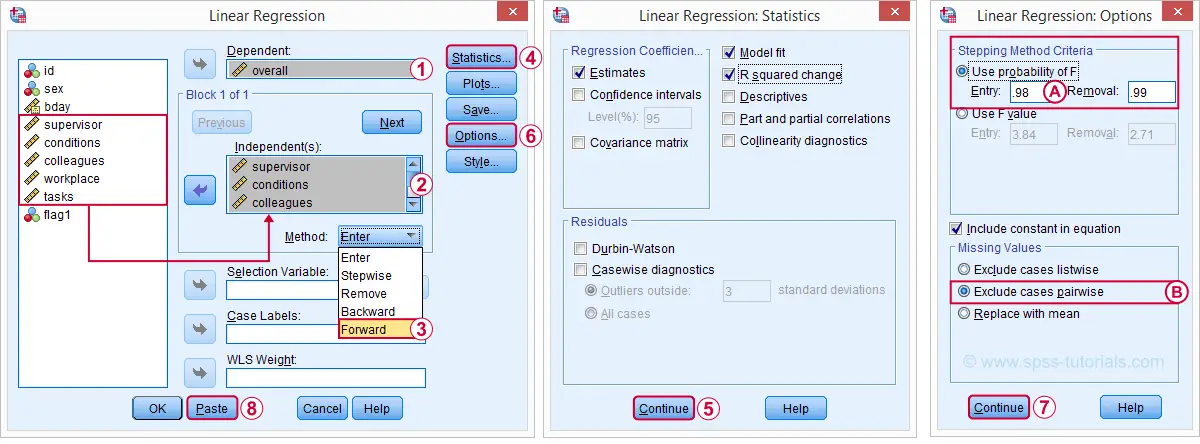The Forward method we chose means that SPSS will all predictors (one at the time) whose p-valuesPrecisely, this is the p-value for the null hypothesis that the population b-coefficient is zero for this predictor. are less than some chosen constant, usually 0.05.Choosing 0.98 -or even higher- usually results in all predictors being added to the regression equation.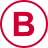By default, SPSS uses only cases without missing values on the predictors and the outcome variable (“listwise deletion”). If missing values are scattered over variables, this may result in little data actually being used for the analysis. For cases with missing values, pairwise deletion tries to use all non missing values for the analysis.Pairwise deletion is not uncontroversial and may occasionally result in computational problems.

## Syntax Regression I - Model Selection

*Regression I: see which model seems right.

REGRESSION
/MISSING PAIRWISE /*... because LISTWISE uses only complete cases...*/
/STATISTICS COEFF OUTS R ANOVA CHANGE
/CRITERIA=PIN(.98) POUT(.99)
/NOORIGIN
/DEPENDENT overall
/METHOD=FORWARD supervisor conditions colleagues workplace tasks.

## Results Regression I - Model Summary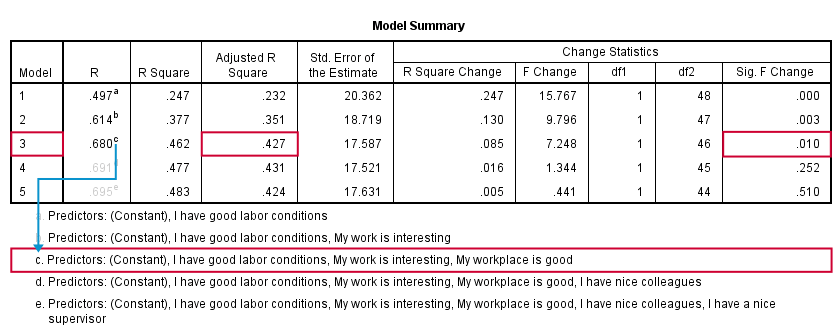SPSS fitted 5 regression models by adding one predictor at the time. The model summary table shows some statistics for each model. The adjusted r-square column shows that it increases from 0.351 to 0.427 by adding a third predictor.
However, r-square adjusted hardly increases any further by adding a fourth predictor and it even decreases when we enter a fifth predictor. There's no point in including more than 3 predictors in or model.
The Sig. F Change column confirms this: the increase in r-square from adding a third predictor is statistically significant, F(1,46) = 7.25, p = 0.010. Adding a fourth predictor does not significantly improve r-square any further. In short, this table suggests we should choose model 3.

## Results Regression I - B Coefficients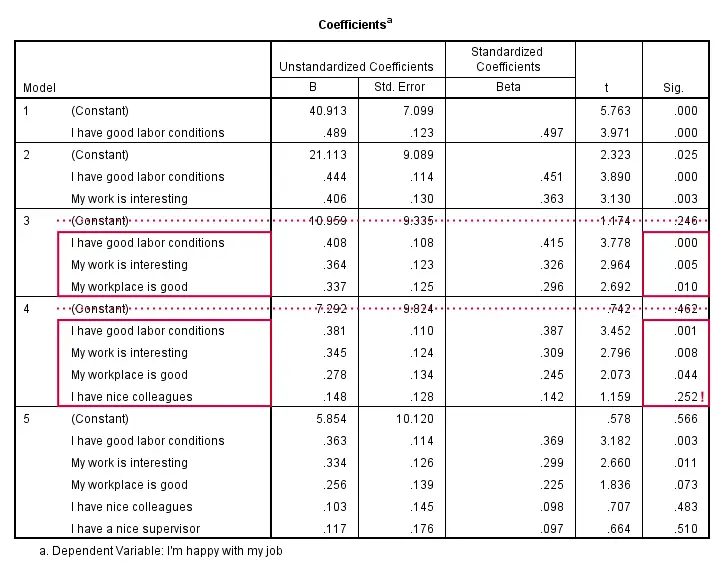The coefficients table shows that all b coefficients for model 3 are statistically significant. For a fourth predictor, p = 0.252. Its b-coefficient of 0.148 is not statistically significant. That is, it may well be zero in our population. Realistically, we can't take b = 0.148 seriously. We should not use it for predicting job satisfaction. It's not unlikely to deteriorate -rather than improve- predictive accuracy except for this tiny sample of N = 50.
Note that all b-coefficients shrink as we add more predictors. If we include 5 predictors (model 5), only 2 are statistically significant. The b-coefficients become unreliable if we estimate too many of them.
A rule of thumb is that we need 15 observations for each predictor. With N = 50, we should not include more than 3 predictors and the coefficients table shows exactly that. Conclusion? We settle for model 3.
So what exactly is model 3? Well, it says that predicted job satisfaction = 10.96 + 0.41 * conditions + 0.36 * interesting + 0.34 * workplace. This formula allows us to COMPUTE our predicted values in SPSS -and the exent to which they differ from the actual values, the residuals. However, an easier way to obtain these is rerunning our chosen regression model. Inspecting them tells us to what extent our regression assumptions are met.

## Regression II - Residual Plots

Let's reopen our regression dialog. An easy way is to use the dialog recall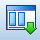tool on our toolbar. Since model 3 excludes supervisor and colleagues, we'll remove them from the predictors box (which -oddly- doesn't mention “predictors” in any way).
Now, the regression procedure can create some residual plots but I rather create them myself. This puts me in control and allows for follow-up analyses if needed. I therefore standardized predicted values and standardized residuals.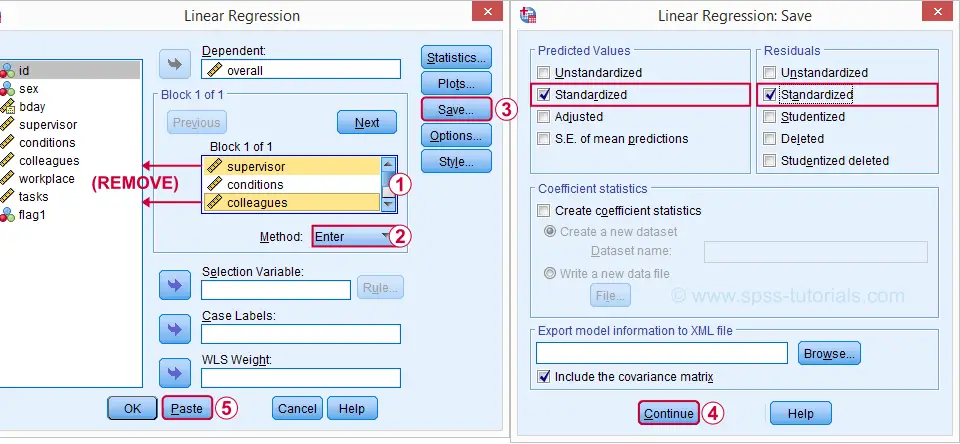## Syntax Regression II - Residual Plots

*Regression II: refit chosen model and save residuals and predicted values.

REGRESSION
/MISSING PAIRWISE
/STATISTICS COEFF OUTS CI(95) R ANOVA CHANGE /*CI(95) = 95% confidence intervals for B coefficients.*
/CRITERIA=PIN(.98) POUT(.99)
/NOORIGIN
/DEPENDENT overall
/METHOD=ENTER conditions workplace tasks /*Only 3 predictors now.*
/SAVE ZPRED ZRESID.

## Results Regression II - Normality Assumption

First note that SPSS added two new variables to our data: ZPR_1 holds z-scores for our predicted values. ZRE_1 are standardized residuals.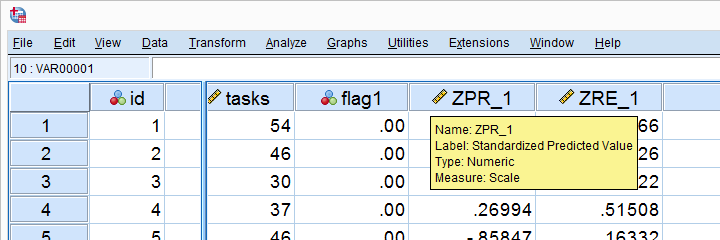Let's first see if the residuals are normally distributed. We'll do so with a quick histogram.

*Histogram for inspecting if residuals are normally distributed.

frequencies zre_1
/format notable
/histogram.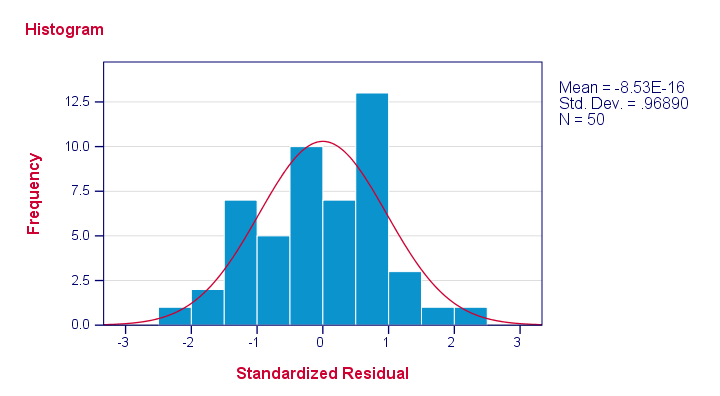If we close one eye, our residuals are roughly normally distributed. Note that -8.53E-16 means -8.53 * 10-16 which is basically zero. I'm not sure why the standard deviation is not (basically) 1 for “standardized” scores but I'll look that up some other day.

## Results Regression II - Linearity and Homoscedasticity

Let's now see to what extent homoscedasticity holds. We'll create a scatterplot for our predicted values (x-axis) with residuals (y-axis).

*Scatterplot for heteroscedasticity and/or non linearity.

GRAPH
/SCATTERPLOT(BIVAR)= zpr_1     WITH zre_1
/title "Scatterplot for evaluating homoscedasticity and linearity".

## ResultFirst off, our dots seem to be less dispersed vertically as we move from left to right. That is, the variance -vertical dispersion- seems to decrease with higher predicted values. Such decreasing variance is an example of heteroscedasticity -the opposite of homoscedasticity. This assumption seems somewhat violated but not too badly.
Second, our dots seem to follow a somewhat curved -rather than straight or linear- pattern but this is not clear at all. If we really want to know, we could try and fit some curvilinear models to these new variables. However, as I argued previously, I think it fitting these for the outcome variable versus each predictor separately is a more promising way to go for evaluating linearity.

I think that'll do for now. Some guidelines on reporting multiple regression results are proposed in SPSS Stepwise Regression - Example 2.

# Tell us what you think!

*Required field. Your comment will show up after approval from a moderator.

# THIS TUTORIAL HAS 20 COMMENTS:

•### By Ashoka Ekanayake on April 9th, 2021

Thank you for great support to understand analysis.

•### By Dr. Batool on May 30th, 2021

i need some help

•### By Marian Hista Villacrusis on July 4th, 2021

This is helpful in current study. Thanks for helping me understand the perks of interpreting data!

•### By ALI on January 19th, 2022

Hi all
•